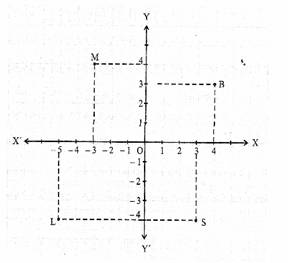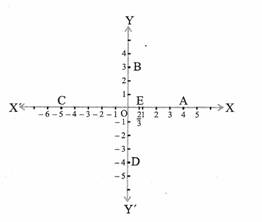# CBSE Important Questions

## Mathmatics Class IX

Q. 1. Answer the following using the given figure :1. The abscissa & the ordinate of the point B are ____________ and ___________. Thus the coordinates of point are _____________
2. The coordinates of the M are __________________
3. What are the X coordinates and Y coordinate of the point B ?

Q. 2. Write the coordinates of the points A, B, C, D and E marked on the given rectangular axes ?Q. 3. Give the answer of the following :-

1. What is the name of horizontal and vertical lines drawn to determine the position of any point in the Cartesian plane ?
2. Write the name of the point where these two lines intersects ?
3. What is the name of each part of the plane formed by these two lines ?

Q. 4. Locate the points (5,0), (0,5), (2,5), (5,2), (-3,5), (-3,-5), (5,-3) & (6,1) in the Cartesian plane ?

Q. 5. In which quadrant do each of the points (-2,4), (3,-1), (-1,0), (1,2) and (-3,-5) lie ? Verify your answer by locating them on the Cartesian plane ?

Q. 6. Plot the points (x,y) given in the following tables on the plane, choosing the suitable units of distance on the axis :

 X -2 -1 0 1 3 Y 8 7 -1.25 3 -1

Q. 7. Answer the following :-

1. How will you locate the position of an object or a point in a plane ?
2. What are the coordinates of origin ?
3. If the abscissa of a point is X & ordinate is Y, then what are the coordinates of that point?

Q. 8. Answer the following :-

1. What are the coordinates of a point on the X axis ?

2. What is the special name given to the plane, used in coordinate Geometry ?

3. What is the distance of a point from the Y axis as well as from the X axis known as ?